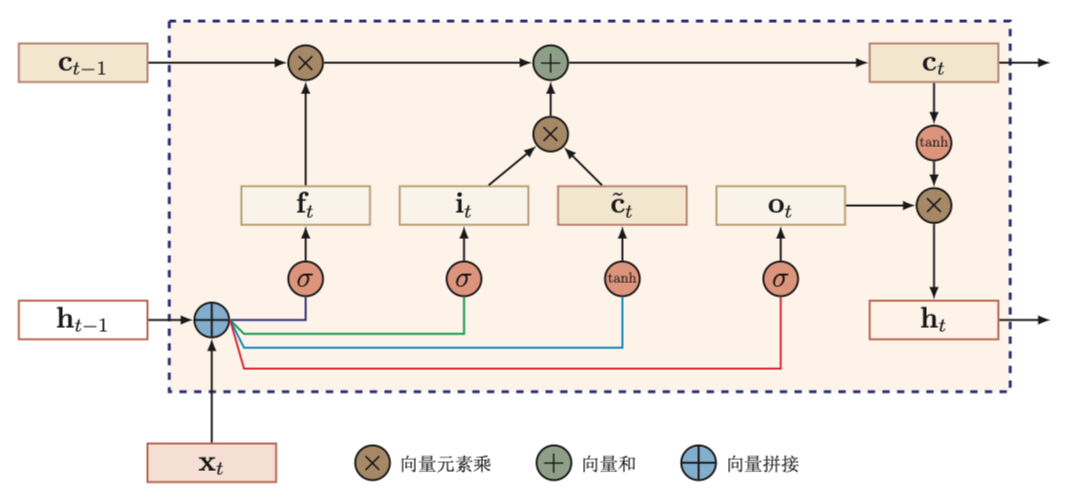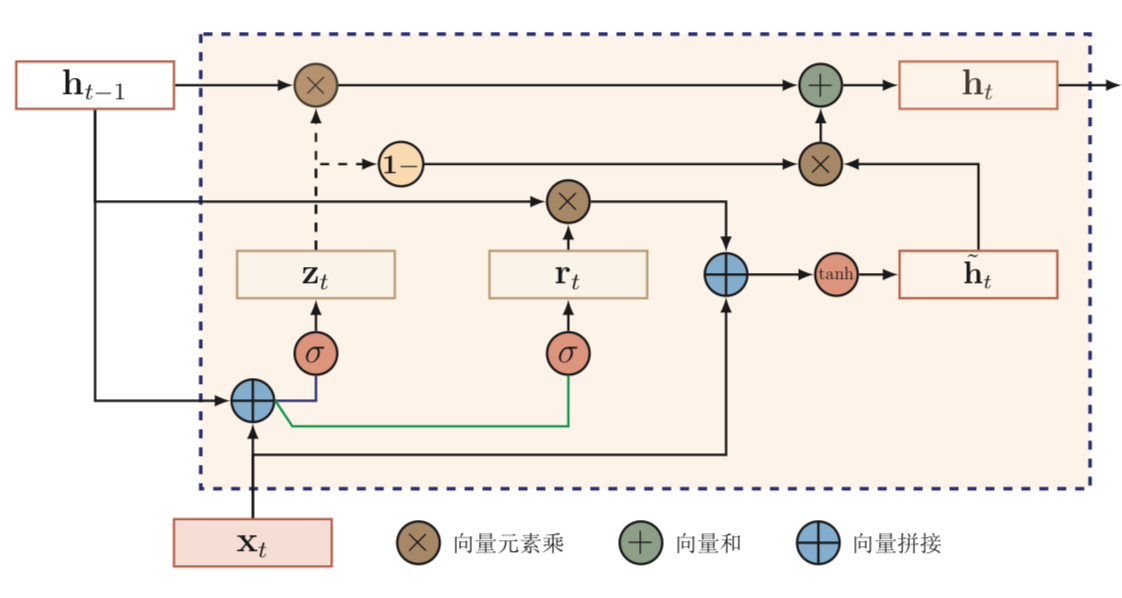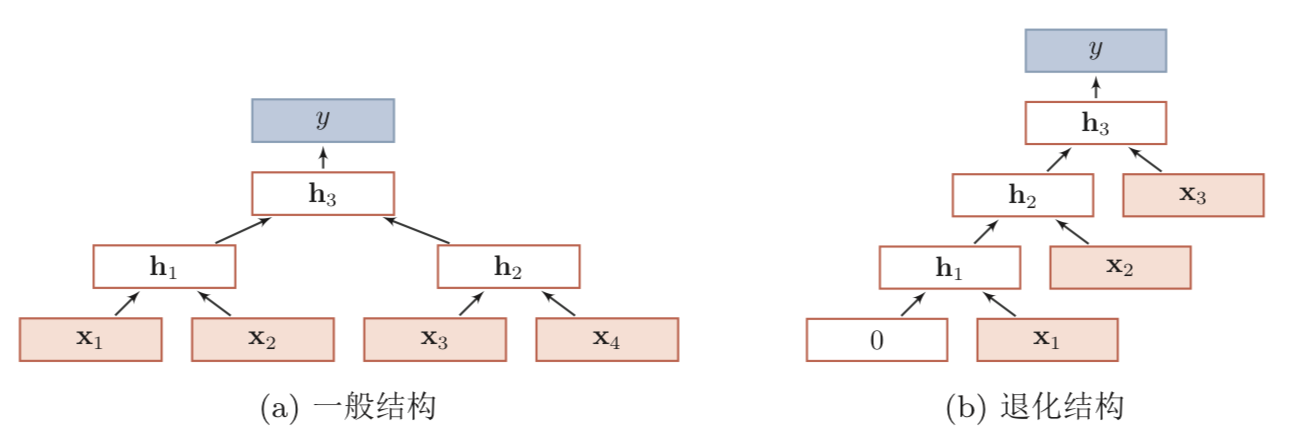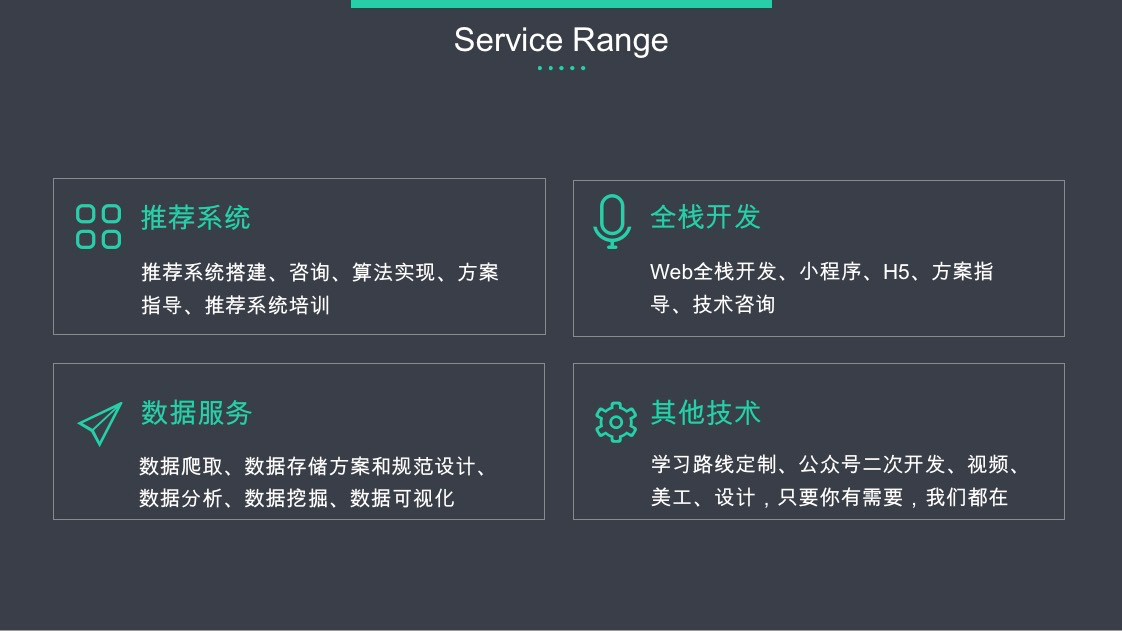Github：https://github.com/thinkgamer

### 引言

• 长短期记忆网络（LSTM）
• 门控循环单元网络（GRU）
• 递归循环神经网络（RecNN）
• 图网络（GN）

### LSTM

LSTM的改进包含两点：

• 新的内部状态
• 门机制

#### 新的内部状态

LSTM网络引入一个新的内部状态（internal state）$c_t$专门进行线性的循环传递，同时（非线性）输出信息给隐藏层的外部状态$h_t$（公式3-1）。

#### 门机制

LSTM网络引入门机制来控制信息的传递， $f_t，i_t，o_t$分别为遗忘门，输入门，输出门。电路中门是0或1，表示关闭和开启，LSTM网络中的门是一种软门，取值在(0,1)，表示以一定比例的信息通过，其三个门的作用分别为：

• $f_t$：控制上一个时刻的内部状态 $c_{t-1}$需要遗忘多少信息
• $i_t$：控制当前时刻的候选状态$\tilde{c_t}$有多少信息需要保存
• $o_t$：控制当前时刻的状态$c_t$有多少信息需要输出为$h_t$

1. 利用当前时刻的输入$x_t$和上一时刻的外部状态$h_{t-1}$计算出三个门和候选状态$\tilde{c_t}$
2. 结合遗忘门$f_t$和输入门$i_t$来更新记忆单元$c_t$
3. 结合输出门$o_t$将内部状态信息传递给外部状态$h_t$### GRU

GRU网络隐状态$h_t$的更新方式为（公式3-7）：

• 若$z_t=0$，当前状态$h_t$和历史状态$h_{t-1}$之间为非线性函数。
• 若$z_t=0，r=1$，GRU退化为简单循环网络
• 若$z_t=0，r=0$，当前状态$h_t$只和当前输入$x_t$有关，和历史状态$h_{t-1}$无关
• 若$z_t=1$，当前时刻状态$h_t=h_{t-1}$，和当前输入$x_t$无关

GRU网络循环单元结构如下（图3-2）：### RecNN### GN

【技术服务】，详情点击查看： https://mp.weixin.qq.com/s/PtX9ukKRBmazAWARprGIAg